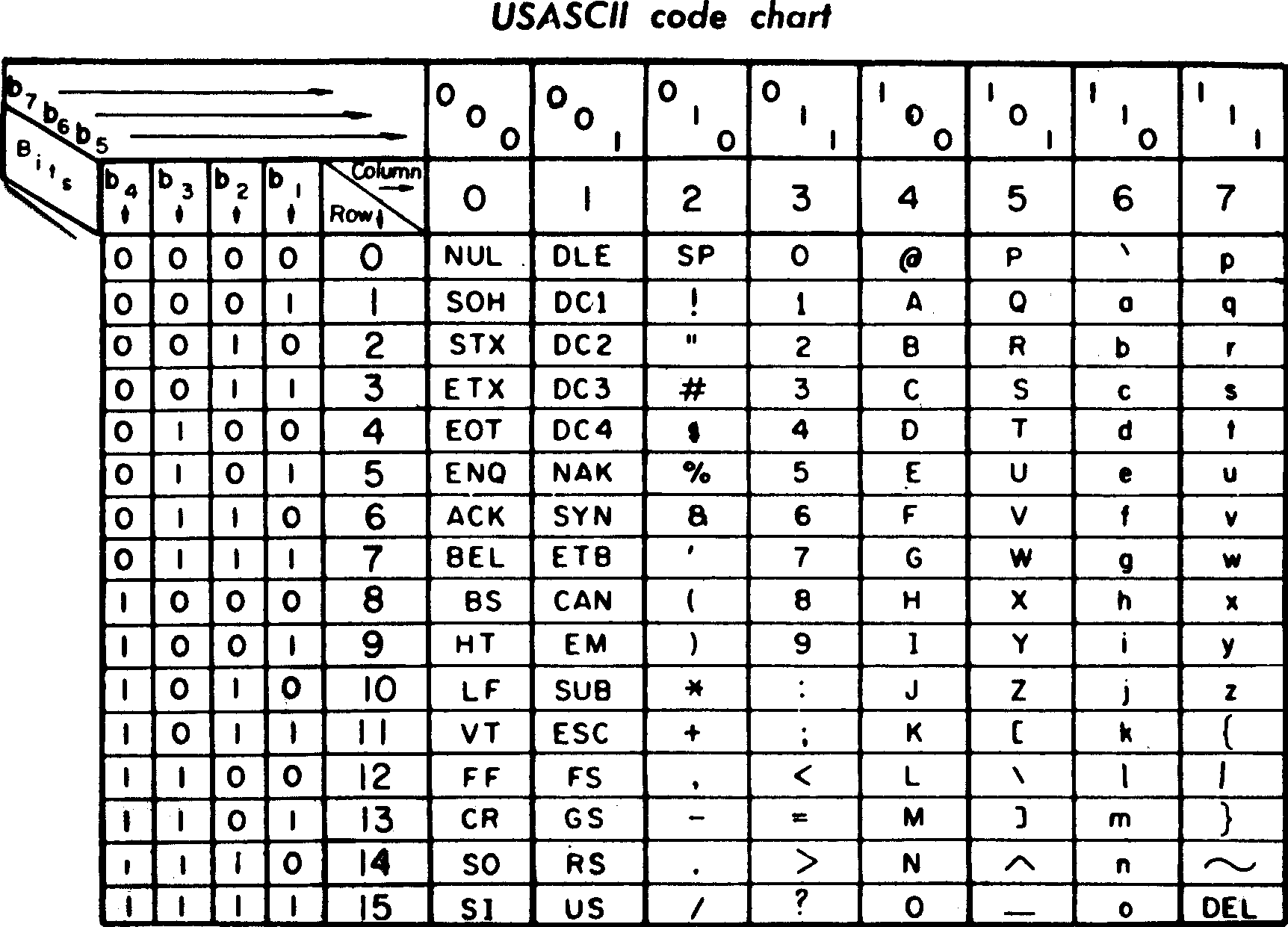# {Updated} ASCII Values & Table Generator in C, What are ASCII values? Why are they needed?

We are all aware of the fact that all computers understand is 0 & 1 – and everything else is stored as patterns containing 0’s and 1’s. Some of us also know that numbers like 25, 12, etc can be represented as patterns of 0’s & 1’s. The computer interprets 25 as 11001 and 12 as 1100.  But how does it interpret a  sentence like “My name is K” or a word like “name” or a letter like “K”? Also, how does it know the difference between ‘k’ & ‘K’?## What are ASCII values? Why are they needed?

This is where ASCII values come in. Each character (including a space) has a certain corresponding number associated to it. And there are different numbers assigned to the lower and upper case of the same alphabet.  For instance, the letter ‘a’ corresponds to the number 97 while ‘A’ corresponds to the number 65.

An ASCII table maps the characters to the numbers. Apart from the equivalent decimal representation, it also gives the hexadecimal and octal representations of the character.

This is what the ASCII table looks like –Here’s a little tool that can help you experiment with ASCII values.

## What’s it got to do with programming?

Now, the more important question. What has your programming got to do with ASCII values?
To begin with, have a look at the following C code –

main()
{
char c;
printf(“Enter any character\n“);
scanf(“%c“, &c); /*scans a character */
printf(“%d“, c); /* prints a decimal */
getch();
}

It scans a character and returns an integer. Do you think the code is incorrect? Try compiling it. It does return a number for every character. And what is this number? Exactly, the decimal ASCII value of the character. Don’t believe me? That’s what the table is for.

Note that %d is responsible for giving us the corresponding decimal value from the ASCII table. We could similarly use %x to hexadecimal and %o for octal values corresponding to the character.

Now, we could try doing it the other way round. Input a number and get the corresponding character, that is.

main()
{
int d;
printf(“Enter any number\n“);
scanf(“%d“, &d); /* scans a decimal */
printf(“%c“, d); /* prints a character */
getch();
}

That’s not it. We may even generate the complete column for a character from the ASCII table. All we need to do is print the dec, hex and oct equivalents for the input character.

main()
{
char c;
printf(“Enter any character\n“);
scanf(“%c“, &c); /*scans a character */
printf(“%d\t“, c); /* prints dec */
printf(“%x\t“, c); /* prints hex */
printf(“%o“, c); /* prints oct */
getch();
}

Now, we can easily use a loop to generate the complete ASCII table. The above table ends at the dec value 127. However, the extended ASCII table is defined to the decimal value 255(ie – it has 256 values).

#include <stdio.h>
#include <conio.h>
/* program to generate the complete ASCII table */
main()
{
int x;
for(x=1;x<=255;x++)
{
printf(“\n%c\t“, x); /* prints char */
printf(“%d\t“, x); /* prints dec */
printf(“%x\t“, x); /* prints hex */
printf(“%o“, x); /* prints oct */
}
getch();
}

You can now try experimenting with ASCII values, decimal, hex, octal and characters. Good Luck! {Btw, Just to let you know – ASCII stands for American Standard Code for Information Interchange}